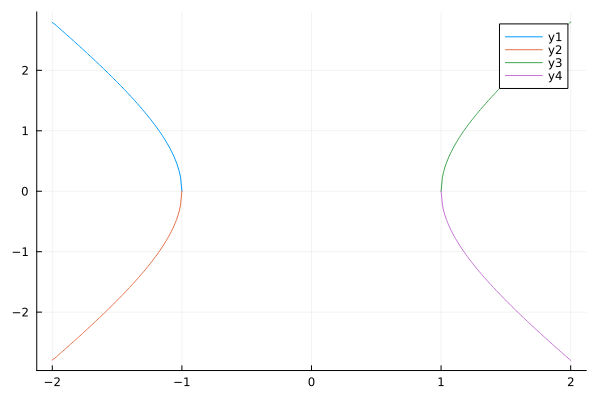# Trying to translate Matlab code to Julia - need help #50640

I’m trying to translate the following code in Matlab:

``````e = 1.05;          %Eccentricity
a = -147562.72;    %km
b = abs(a)*sqrt(e.^2 -1);      %Equation for Semi-Minor Axis, b

x1 = linspace(   a, 2*a, 1E3);         %From 0 to a, Upper

ytop =  b*sqrt(x1.^2/a^2-1);  %Upper Hyperbola Part
ybot = -b*sqrt(x1.^2/a^2-1);  %Lower Hyperbola Part
``````

to Julia.

Everything seems to go well until `ytop`. Here’s my code up to that point:

`````` ecc=1.05; a=-14; b=abs(a)*sqrt(ecc.^2 -1)

x1=LinRange(a, 2*a, 100)
100-element LinRange{Float64, Int64}:
-14.0,-14.1414,-14.2828,-14.4242,-14.5657,…,-27.5758,-27.7172,-27.8586,-28.0
``````

but then:

`````` ytop=b*sqrt(x1.^2 / a^2 - 1)
ERROR: MethodError: no method matching -(::Vector{Float64}, ::Int64)
For element-wise subtraction, use broadcasting with dot syntax: array .- scalar
Closest candidates are:
-(::T, ::T) where T<:Union{Int128, Int16, Int32, Int64, Int8, UInt128, UInt16, UInt32, UInt64, UInt8} at ~/julia-1.7.1/share/julia base/int.jl:86
-(::Rational, ::Integer) at ~/julia-1.7.1/share/julia/base/rational.jl:311
-(::T, ::Integer) where T<:AbstractChar at ~/julia-1.7.1/share/julia/base/char.jl:227
...
Stacktrace:
 top-level scope
@ REPL:1
``````

I went through Julia and Matlab docs trying to understand the Error message (making several changes to the code, trying to match the types, for example), but I couldn’t do it.

Please forgive me my probable ignorance (both of Julia and Matlab), and be willing to help me please.

You need to broadcast the operations with a “.” as hinted to by the error message ` use broadcasting with dot syntax: array .- scalar`

``````ytop=b*sqrt.(x1.^2 / a^2 .- 1)
``````
1 Like

In Matlab, a few operations have been identified as doing different things if applied as a scalar operation elementwise or as a matrix-matrix operation. In these cases you mark the difference with a period: `A * x` vs `A .* x`. In Julia, this distinction is applied consistently, and broadcasting (“elementwising”) can and is expected to be done for any function. For instance, if you define a function `f(x) = sin(x)*x^2`, you cannot now call `f` on a matrix `X`, but you can broadcast it: `f.(X)`.

It takes a while to get used to, but it is really convenient, because you can easily do things like “sum every array in this array of arrays”, with quite intuitive syntax.

2 Likes

Small correction: actually you can, but by default the `sin`, `*` and `^2` will be interpreted in a matrix-algebraic sense (i.e. `sin` is defined as the Taylor expansion). On the other hand, that function `f` wouldn’t work for vectors as is.

``````julia> f(x) = sin(x) * x^2
f (generic function with 1 method)

julia> A = rand(2, 2)
2×2 Matrix{Float64}:
0.42875   0.743563
0.466328  0.963168

julia> x = rand(2)
2-element Vector{Float64}:
0.3776789845468388
0.49083688309729057

julia> f(A)
2×2 Matrix{Float64}:
0.515227  1.00944
0.633075  1.24074

julia> f.(A)
2×2 Matrix{Float64}:
0.0764227  0.374257
0.0977732  0.761641

julia> f(x)
ERROR: MethodError: no method matching sin(::Vector{Float64})
...

julia> f.(x)
2-element Vector{Float64}:
0.052601022788648956
0.11356144554205107
``````

Note how `f.(A)` is different from `f(A)`

2 Likes

Ah, yes. You cannot call them on arbitrary matrices, only ones for which sine and square are defined.

``````ytop = b .* sqrt.(x1.^2 ./ a^2 .- 1)
``````

This dots everything except `a^2`, which is a scalar-scalar operation.

1 Like

And you can automatically broadcast everything in an expression with the `@.` macro:

``````ytop = @. b * sqrt(x1^2 / a^2 - 1)
``````
2 Likes

Indeed, but for optimal performance, you would like to avoid dotting `a^2`.

I have not used this before, but this claims to be a Matlab-to-Julia translator.
If it works, I hope all Matlab users run this on their code and are happy with the results.

1 Like
``````@btime ytop=b*sqrt.(x1.^2 / a^2 .- 1)
@btime ytop1=b.*sqrt.(x1.^2 ./ a^2 .- 1)
@btime ytop2= @. b*sqrt(x1^2 / a^2 - 1)
``````

gives

1.930 μs (14 allocations: 3.84 KiB)
2.756 μs (14 allocations: 1.66 KiB)
3.200 μs (18 allocations: 1.86 KiB)

(Julia 1.8.5)

Always better to wrap your test articles in functions for benchmarking:

``````julia> f1(x1, a, b) = b .* sqrt.(x1.^2 ./ a^2 .- 1)
f1 (generic function with 1 method)

julia> f2(x1, a, b) = @. b * sqrt(x1^2 / a^2 - 1)
f2 (generic function with 1 method)

julia> @btime f1(\$x1, \$a, \$b);
4.143 μs (1 allocation: 7.94 KiB)

julia> @btime f2(\$x1, \$a, \$b);
4.143 μs (1 allocation: 7.94 KiB)
``````

At least on Julia v1.9.2, the generated assembly is identical - the scalar doesn’t get broadcasted.

2 Likes

Ah, it’s clever enough to avoid that extra operation, interesting.

Thank you all

The first solution was enough to solve my problem, but I will go through all the others (and even find a more elegant way to plot it).
For now the plot is:

``````p = plot(x1,ytop); plot!(p,x1,ybot); plot!(p,-x1,ytop); plot!(p,-x1,ybot)
``````P.S.: I was pleasantly surprised to find such a lively community here. I guess I’ll have to take some time to understand better broadcasting process in Julia.

1 Like

This blog post from 2017 (when the dot-broadcasting syntax was first introduced in Julia v0.6) does a really nice job of explaining how/why it all works, if you’re interested in reading more:

1 Like

JuliaAcademy is a great resource, even for experienced programmers. As a matter of fact, the Introduction to Julia (for programmers) course lesson on Functions discusses Map and Broadcast (very similar).

The courses do help to explain differences Julia and other languages. It’s worth looking at in your spare time.I’d also like to give a shout out and THANK YOUto all of the creators and maintainers for JuliaAcademy!!